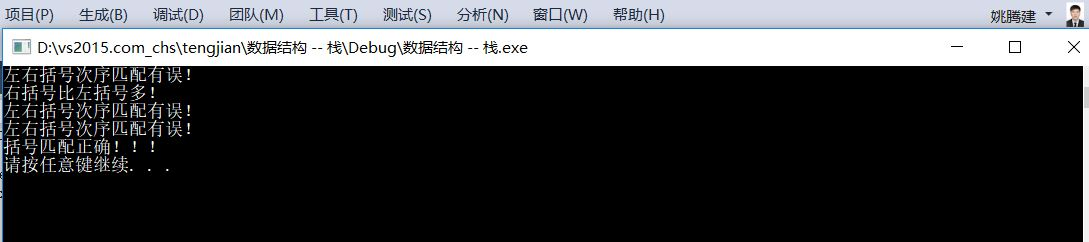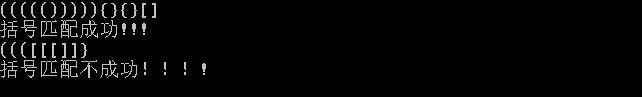括号配对数据结构_数据结构实验之栈与队列四:括号匹配 测试数据 - CSDN
• 给定一个字符串，其中的字符只包含三种括号：花括号{ }、中括号[ ]、圆...括号匹配要求括号必须以正确的顺序配对，如“{ [ ] ( ) }”或 “[ ( { } [ ] ) ]” 等为正确的格式，而“[ ( ] )”或“{ [ ( ) }”或“( { ...
       给定一个字符串，其中的字符只包含三种括号：花括号{ }、中括号[ ]、圆括号( )，即它仅由 “( ) [ ] { }” 这六个字符组成。设计算法，判断该字符串是否有效，即字符串中括号是否匹配。括号匹配要求括号必须以正确的顺序配对，如 “{ [ ] ( ) }” 或 “[ ( { } [ ] ) ]” 等为正确的格式，而 “[ ( ] )” 或 “{ [ ( ) }” 或 “( { } ] )” 均为不正确的格式。

这个问题可以用栈stack来解决，具体的代码如下：

#pragma once

#include<stdio.h>
#include<assert.h>
#include<string.h>

//typedef int DataType;
typedef char DataType;

#define MAX_SIZE 100

typedef struct Stack
{
DataType _arr[MAX_SIZE];
int _top;
}Stack;

void StackInit(Stack* s)
{
assert(s);
s->_top = 0;
}

int StackEmpty(Stack* s)
{
assert(s);
return 0 == s->_top;
}

void StackPush(Stack* s, DataType data)
{
assert(s);
if (s->_top == MAX_SIZE)
{
printf("栈已经满了！\n");
}
s->_arr[s->_top] = data;
s->_top++;
}
void StackPop(Stack* s)
{
assert(s);
if (StackEmpty(s))
{
printf("栈已经空了！\n");
return;
}
s->_top--;

}
DataType StackTop(Stack* s)
{
assert(s);
return s->_arr[s->_top - 1];
}
int StackSize(Stack* s)
{
assert(s);
return s->_top;
}

//
void Test()
{
Stack s;
StackInit(&s);

StackPush(&s, 1);
StackPush(&s, 2);
StackPush(&s, 3);
StackPush(&s, 4);
StackPush(&s, 5);
StackPush(&s, 6);

printf("size = %d\n", StackSize(&s));
printf("top = %d\n", StackTop(&s));

StackPop(&s);
printf("size = %d\n", StackSize(&s));
printf("top = %d\n", StackTop(&s));
}

括号匹配/
int isBracket(char ch)
{
if (('(' == ch || ')' == ch) ||
('[' == ch || ']' == ch) ||
('{' == ch || '}' == ch))
return 1;
return 0;
}
int MatchBrackets(const char* pStr)
{
int len = 0, i = 0;
Stack s;
if (NULL == pStr)
{
return 1;
}
StackInit(&s);
len = strlen(pStr);
for (; i < len; ++i)
{
if (isBracket(pStr[i]))
{
if ('(' == pStr[i] || '[' == pStr[i] || '{' == pStr[i])
{
StackPush(&s, pStr[i]);
}
else
{
if (StackEmpty(&s))
{
printf("右括号比左括号多！\n");
return 0;
}
else
{
//用当前的括号和栈顶元素匹配
char top = StackTop(&s);
if ('(' == top && ')' == pStr[i] ||
'[' == top && ']' == pStr[i] ||
'{' == top && '}' == pStr[i])
{
StackPop(&s);
}

else
{
printf("左右括号次序匹配有误！\n");
return 0;
}

}
}
}
}
if (!-StackEmpty(&s))
{
printf("左括号比右括号多！\n");
return 0;
}

printf("括号匹配正确！！！\n");
return 1;
}

void TestMatchBrackets()
{
char a[] = "(())abc{[(])}";
char b[] = "(()))abc{[]}";
char c[] = "(()()abc{[]}";
char d[] = "(())abc{[]()}";
char e[] = "{}";
MatchBrackets(a);
MatchBrackets(b);
MatchBrackets(c);
MatchBrackets(d);
MatchBrackets(e);

}

#include "Stack.h"
int main()
{
TestMatchBrackets();
system("pause");
return 0;
}

以上是用C语言对栈和括号匹配问题的简单实现，代码结果如下：展开全文数据结构 括号匹配 算法
• 请编写一个程序检查表达式中的左右圆括号是否匹配，若匹配，则返回“YES”；否则返回“NO”。表达式长度小于255，左圆括号少于20个。 输入格式 一行：表达式 输出格式 一行：“YES” 或“NO” 输入输出样例 输入 #1 ...
题目描述
假设一个表达式有英文字母（小写）、运算符（+，—，*，/）和左右小（圆）括号构成，以“@”作为表达式的结束符。请编写一个程序检查表达式中的左右圆括号是否匹配，若匹配，则返回“YES”；否则返回“NO”。表达式长度小于255，左圆括号少于20个。
输入格式
一行：表达式
输出格式
一行：“YES” 或“NO”
输入输出样例
输入 #1 复制
2*(x+y)/(1-x)@
输出 #1 复制
YES
输入 #2 复制
(25+x)(a(a+b+b)@
输出 #2 复制
NO
说明/提示
表达式长度小于255，左圆括号少于20个
#include<iostream>
#include<algorithm>
#include <stack>
using namespace std;
stack<char> a;
int main()
{
char str;
int i=0;
while(cin>>str&&str!='@')
{
if(a.empty()==1&&str==')')
{
a.push(str);
break;
}
else if(str=='(')
a.push(str);
else if(str==')'&&a.empty()==0)
{
if(a.top()=='(')
a.pop();
}
else
continue;
}
if(a.empty()==1)
cout<<"YES"<<endl;
else
cout<<"NO"<<endl;
}



展开全文• 问题： 输入一个带有 '(' 、')'的字符串，编写程序判断左右括号个数是否匹配。 输入：（4+3）*5/（（34+2）-64） 思路： | 若有左括号压入栈中 输入字符串--->遍历各字符---> | ...
问题：

输入一个带有 '(' 、')'的字符串，编写程序判断左右括号个数是否匹配。

输入：（4+3）*5/（（34+2）-64）

思路：

|  若有左括号压入栈中

输入字符串--->遍历各字符--->   |                                                                            --->判断栈是否为空--->空--->匹配

|  若有右括号进入判断--->栈为空--->括号不匹配                                --->不空--->不匹配

--->栈不空--->将栈顶弹出

c++代码：

#include<stack>
#include<string>
#include<iostream>

using namespace std;
bool matchParenthesis(const string& s)
{
stack<char> let;
string::size_type len = s.length();
for(string::size_type i=0;i<len;i++)
{
char a = s.at(i);
if(a=='(')
let.push(a);
else if(a==')')
{
if(let.empty())
{
cout<<"false"<<endl;
return false;
}
let.pop();
}
}
if(let.empty())
{
cout<<"true"<<endl;
return true;
}
else
{
cout<<"false"<<endl;
return false;
}
}
int main(void)
{
string s;
cin>>s;
matchParenthesis(s);
}


展开全文括号匹配
• win32控制台应用程序 vs2010以上编译通过 输入含（）{}[]的表达式 判断括号是否正确匹配 大连理工大学软件学院数据结构上机题c++ 数据结构
• 解决办法：用c++stl中提供的数据结构stack 分析：用一个字符串保存输入的表达式，去遍历字符串，将字符串中的‘（’ 、‘ [ ’、‘ {’ 三个字符压入栈中，当遇到右括号时取出栈顶元素与之比较，若不匹配，则整个...
题目要求：判断含（），[ ],{ }的算术表达式中所有的括号是否正确匹配。
解决办法：用c++stl中提供的数据结构stack
分析：用一个字符串保存输入的表达式，去遍历字符串，将字符串中的‘（’ 、‘ [ ’、‘ {’ 三个字符压入栈中，当遇到右括号时取出栈顶元素与之比较，若不匹配，则整个字符串中的括号是不匹配的。遇到其它字符时不用处理。
#include<iostream>
#include<cstdio>
#include<string>
#include<stack>
using namespace std;
int main()
{
string s;
stack<char> mystack;
while (cin >> s) {
for (auto c : s)
{
if (c == '(' || c == '{' || c == '[') mystack.push(c);
if (c == '}' || c == ']')
{
if (!mystack.empty() && mystack.top() == (c - 2)) mystack.pop();   //ASCII码中[]和{}括号差二
else
{
cout << "括号匹配不成功！！！!" << endl;
return 0;
}
}
if (c == ')')
{
if (!mystack.empty() && mystack.top() == (c - 1)) mystack.pop();  //ASCII码中（）括号差一
else
{
cout << "括号匹配不成功！！！!" << endl;
return 0;
}
}
}
if (mystack.empty())
cout<<"括号匹配成功!!!"<<endl;
else
cout<<"左括号多余"<<endl;
}
return 0;
}

解释一下，方括号和花括号的ASCII码右括号比左括号大2，圆括号的右括号比左括号大1.
运行结果：展开全文• 每当读入一个右括号，若与当前栈顶的左括号类型相同，则二者匹配，将栈顶的左括号出栈，直到表达式扫描完毕在处理过程中，还要考虑括号匹配出错的情况。 2.算法步骤 ①初始化一个空栈S ②设置一标记性变flag，...
• 输入一包含（和）的字符串，检测括号是否匹配(其中括号能嵌套括号），并输出括号是否匹配的信息（匹配，缺少左括号，缺少右括号）。栈的应用
• 括号匹配（C++数据结构） 描述：给出由()、{}、[]三种类型括号所组成的字符串，判断所给字符串中的括号能否全部匹配,若能匹配，则输出true！，否则输出false！ 输入输出示例 输入 ({}) ([{]) 输出 true! ...数据结构 C++
• 基于数据结构括号匹配问题，有源代码，文档，执行文件等
• 问题描述：对一个字符串的左右括号进行匹配。 输入：一个字符串表达式 输出：匹配成功true或匹配失败false 例如：假设一个算术表达式中可以包含三种括号：圆括号“()”，方括号“[]”和花括号“{}”，且这三种...括号匹配
• 括号匹配的检验: eg: [([][][)]] 不匹配 [([][])] 匹配思路： 0x0.首先建立两个栈，并对其初始化 0x1.对表达式进行遍历，将相邻两个不能匹配的入栈到栈A，然后检测栈空间A是否为偶数，如果是表明有存在的可能，...
• 数据结构实验之栈与队列四：括号匹配 Time Limit:1000 msMemory Limit:65536 KiB SubmitStatistic Problem Description 给你一串字符，不超过50个字符，可能包括括号、数字、字母、标点符号、空格，你的任务是...
• 括号匹配算法在各种编程的IDE工具中都会用到，用来检测关于括号匹配的语法错误，括号匹配实际上不复杂，主要就是利用栈这个数据结构，扫描输入的字符串，若遇到左括号则直接入栈，若遇到右括号则弹出栈顶括号，看...数据结构 括号匹配
• 【C++数据结构与算法】括号匹配算法这是从《c++数据结构与程序设计》这本书改过来的一个算法。 会用到c++内置的数据结构栈。要解决的问题：输入一行括号，判断这行括号是否匹配算法原理和代码思路一句话概括算法...c++
• 输入一串字符串，编写算法判断字符串中的括号是否匹配，如果匹配，输出1，否则输出0。 注： 只考虑半角括号：( ) { } [ ]，不考虑全角括号：（ ） 【 】 例如：{ab123[(3*6-(4+3)) {223}hhh} 字符串中的括号...
• 其实匹配问题中主要运用到的数据结构就是栈，例如对一个字符串进的左右括号进行匹配，字符串（a*(b+c)+d）在位置0和位置3有左括号，在位置7和10有右括号，位置0的左括号与位置10的右括号匹配，位置3左括号和位置7...
• 括号配对问题描述： 现在，有一行括号序列，请你检查这行括号是否配对。输入： 第一行输入一个数N（0&lt;N&lt;=100）,表示有N组测试数据。后面的N行输入多组输入数据，每组输入数据都是一个字符串S(S的...
• 数据结构与算法实验3（栈） 括号匹配 用栈ADT应用：对称符号匹配判断 输入一行符号，以#结束，判断其中的对称符号是否匹配。对称符号包括： { } 、 [ ] 、 ( )、 < > 输出分为以下几种情况： ...
• 题目描述： 思路： 代码实现 #ifndef STACK #define STACK typedef struct { char str; int top; }*stack, Stack; #endif #define max 20 #include&lt;stdio.h&...#inc...
• #include #define MAXSIZE 100 typedef int selemtype; ...typedef struct { //顺序栈的存储结构 selemtype *base; selemtype *top; int stacksize; }SqStack; void InitStack(SqStackC++ 数据结构 新手
...